# Posts Tagged ‘ data analysis ’

## Graph wide data and long data in SAS

June 3, 2019
By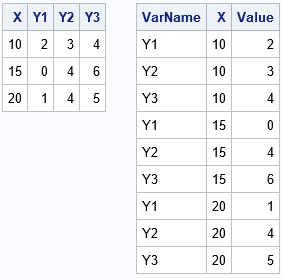Statistical programmers and analysts often use two kinds of rectangular data sets, popularly known as wide data and long data. Some analytical procedures require that the data be in wide form; others require long form. (The "long format" is sometimes called "narrow" or "tall" data.) Fortunately, the statistical graphics procedures

Tags: , ,
Posted in SAS | Comments Off on Graph wide data and long data in SAS

## The Theil-Sen robust estimator for simple linear regression

May 28, 2019
By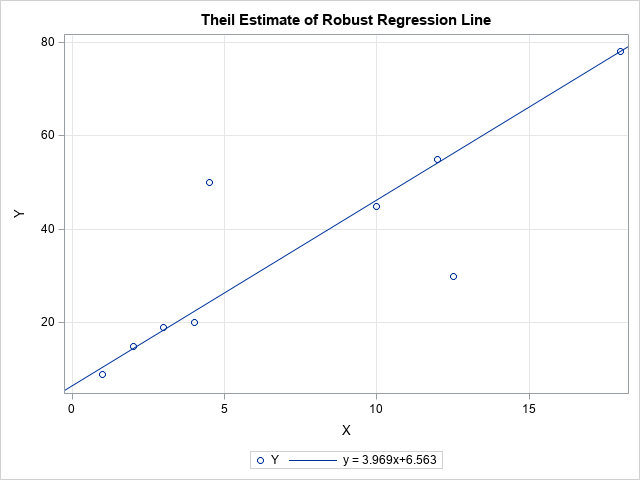Modern statistical software provides many options for computing robust statistics. For example, SAS can compute robust univariate statistics by using PROC UNIVARIATE, robust linear regression by using PROC ROBUSTREG, and robust multivariate statistics such as robust principal component analysis. Much of the research on robust regression was conducted in the

Tags: , ,
Posted in SAS | Comments Off on The Theil-Sen robust estimator for simple linear regression

## What is Kolmogorov’s D statistic?

May 15, 2019
By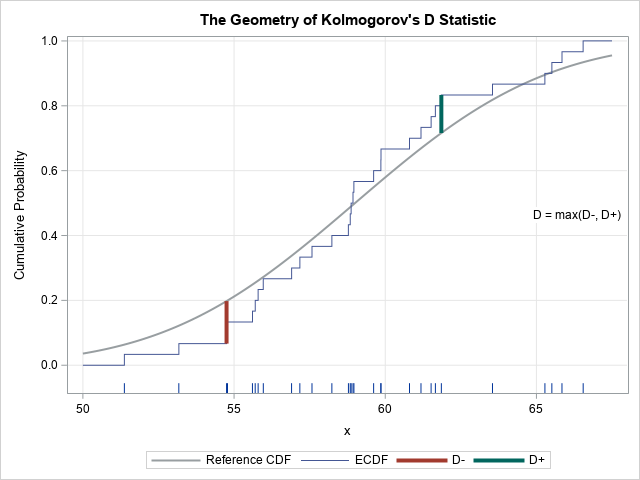Have you ever run a statistical test to determine whether data are normally distributed? If so, you have probably used Kolmogorov's D statistic. Kolmogorov's D statistic (also called the Kolmogorov-Smirnov statistic) enables you to test whether the empirical distribution of data is different than a reference distribution. The reference distribution

Tags: , ,
Posted in SAS | Comments Off on What is Kolmogorov’s D statistic?

## Discrimination, accuracy, and stability in binary classifiers

May 8, 2019
By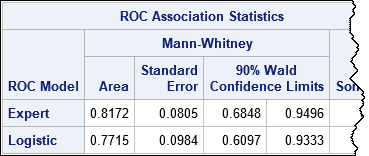At SAS Global Forum 2019, Daymond Ling presented an interesting discussion of binary classifiers in the financial industry. The discussion is motivated by a practical question: If you deploy a predictive model, how can you assess whether the model is no longer working well and needs to be replaced? Daymond

Tags: , , ,
Posted in SAS | Comments Off on Discrimination, accuracy, and stability in binary classifiers

## A CUSUM test for autregressive models

April 24, 2019
By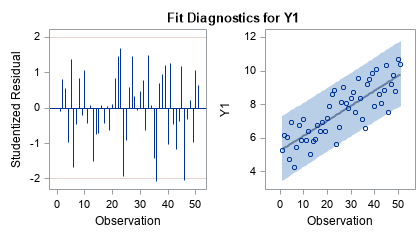The CUSUM test has many incarnations. Different areas of statistics use different assumption and test for different hypotheses. This article presents a brief overview of CUSUM tests and gives an example of using the CUSUM test in PROC AUTOREG for autoregressive models in SAS. A CUSUM test uses the cumulative

Tags: ,
Posted in SAS | Comments Off on A CUSUM test for autregressive models

## The CUSUM test for randomness of a binary sequence

April 22, 2019
By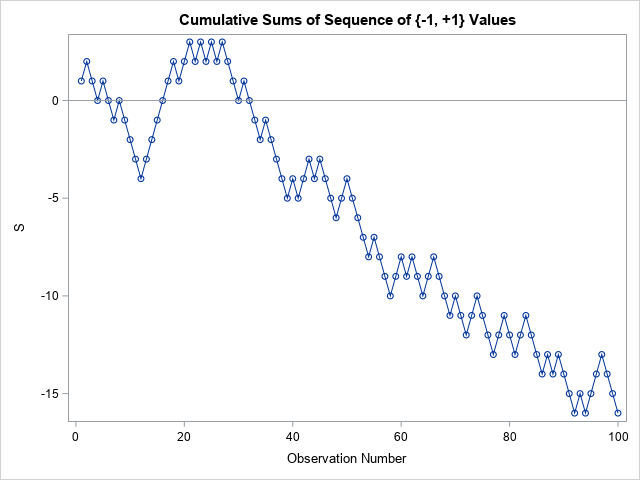Many statistical tests use a CUSUM statistic as part of the test. It can be confusing when a researcher refers to "the CUSUM test" without providing details about exactly which CUSUM test is being used. This article describes a CUSUM test for the randomness of a binary sequence. You start

Tags: , ,
Posted in SAS | Comments Off on The CUSUM test for randomness of a binary sequence

## Convergence in mixed models: When the estimated G matrix is not positive definite

April 3, 2019
By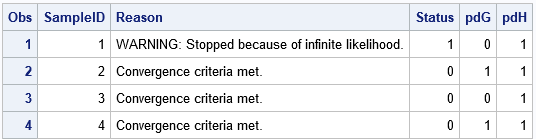I've previously written about how to deal with nonconvergence when fitting generalized linear regression models. Most generalized linear and mixed models use an iterative optimization process, such as maximum likelihood estimation, to fit parameters. The optimization might not converge, either because the initial guess is poor or because the model

Tags: , ,
Posted in SAS | Comments Off on Convergence in mixed models: When the estimated G matrix is not positive definite

## Matrix operations and BY groups

April 1, 2019
By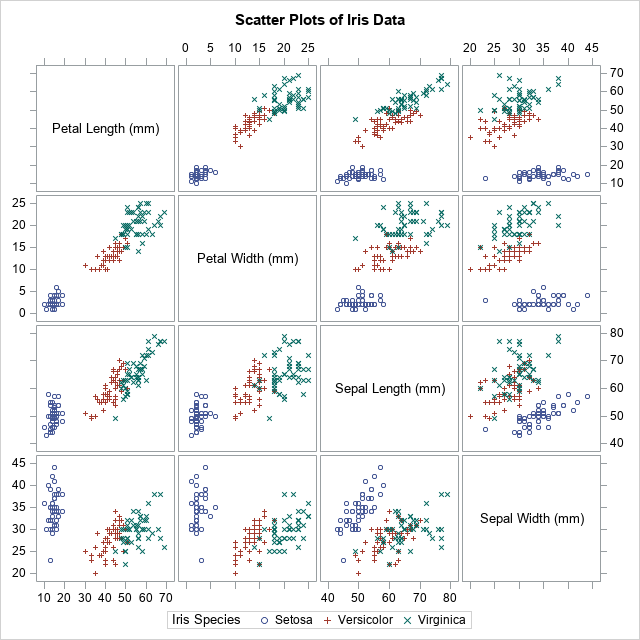Many SAS procedures support the BY statement, which enables you to perform an analysis for subgroups of the data set. Although the SAS/IML language does not have a built-in "BY statement," there are various techniques that enable you to perform a BY-group analysis. The two I use most often are